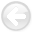Conjugate guided gradient (CGG) method for robust inversion and its application to velocity-stack inversionNext: INTRODUCTION Up: Reproducible Documents

# Conjugate guided gradient (CGG) method for robust inversion and its application to velocity-stack inversion

Jun Ji

Department of Information System Engineering,
Hansung University, Samsun-dong 2-ga, Sungbuk-ku, Seoul, Korea

### Abstract:

This paper proposes a modified conjugate gradient (CG) method, called the conjugate guided gradient (CGG) method, that is a robust iterative inversion method producing a parsimonious model estimation. The CG method for solving least-squares (LS) (i.e.-norm minimization) problems is modified to solve for different norms or different minimization criteria by guiding the gradient vector appropriately during iteration steps. The guiding is achieved by iteratively reweighting either the residual vector or the gradient vector during iteration steps like IRLS (Iteratively Reweighted Least Squares) method does. The robustness is achieved by weighting the residual vector and the parsimonious model estimation is obtained by weighting the gradient vector. Unlike the IRLS method, however, the CGG method doesn't change the corresponding forward operator of the problem and is implemented in a linear inversion template. Therefore the CGG method requires less computation than the IRLS method does. Since the solution in the CGG method is found in a least-squares sense along the gradient direction guided by the weights, the solution found by the CGG method can be interpreted as the LS solution located in the guided gradient direction. Guiding the gradient gives us more flexibility in choice of weighting parameters than the one of the IRLS method. I applied the CGG method to velocity-stack inversion, and the results show that the CGG method gives a far more robust and parsimonious model estimation than the standard-norm solution, with the results comparable to the-norm IRLS solution.Conjugate guided gradient (CGG) method for robust inversion and its application to velocity-stack inversionNext: INTRODUCTION Up: Reproducible Documents

2011-06-26